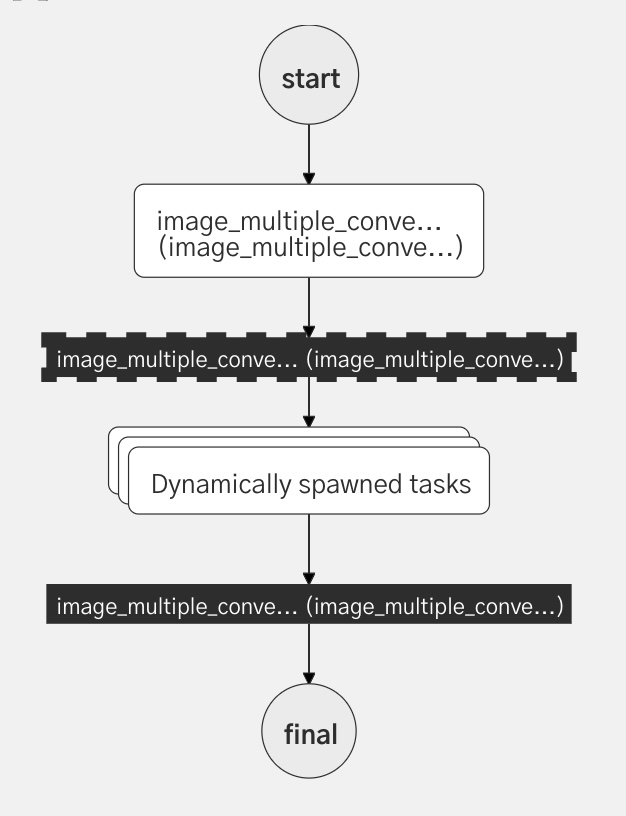``"type" : "FORK_JOIN_DYNAMIC"``
Important Note

This is the legacy method of using a dynamic fork join which requires a preparation task for the inputs. There is a new updated version of this that can operate on an array Dynamic Fork Join using Arrays

## Introduction​

When the number of forks must be determined at run-time, the FORK_JOIN_DYNAMIC task is needed. (In a regular fork operation -the FORK_JOIN task- the number of forks is defined during workflow creation.)

Note:

A `FORK_JOIN_DYNAMIC` can only have one task per fork. If there is a need for multiple tasks per fork, a sub-workflow can be utilized.

## Configuration​

Here is a JSON example of a `FORK_JOIN_DYNAMIC` task followed by a `JOIN` task:

``{  "inputParameters": {    "dynamicTasks": "\${fooBarTask.output.dynamicTasksJSON}",    "dynamicTasksInput": "\${fooBarTask.output.dynamicTasksInputJSON}"  },  "type": "FORK_JOIN_DYNAMIC",  "dynamicForkTasksParam": "dynamicTasks",  "dynamicForkTasksInputParamName": "dynamicTasksInput"},{"name": "image_multiple_convert_resize_join","taskReferenceName": "image_multiple_convert_resize_join_ref","type": "JOIN"}``

Let's look at the `inputParameters` for the `FORK_JOIN_DYNAMIC` task.

• `dynamicForkTasksParam` This is a JSON array that lists the tasks in each fork that is to be created. Each entry corresponds to a separate fork.
• `dynamicForkTasksInputParamName` This is a JSON array where the keys are the taskReferenceName for each fork, and the values are the `inputParameters` for each task.

NOTE: `fooBarTask` is a task that is defined prior to the FORK_JOIN_DYNAMIC in the workflow definition. This task will create the `dynamicTasks` and `dynamicTasksInput` JSON arrays that are required for the DYNAMIC task.

## Input Configuration​

AttributeDescription
nameTask Name. A unique name that is descriptive of the task function
type`FORK_JOIN_DYNAMIC`
inputParametersThe input parameters that will be supplied to this task.
dynamicForkTasksParamThis is a JSON array listing each of the tasks/sub-workflow objects in each fork. (Note: This has a different format for `SUB_WORKFLOW` compared to other tasks.)
dynamicForkTasksInputParamNameA JSON array: keys are task/sub-workflow names for each fork, and the values are the corresponding inputParameters for each task.

## Example​

Note: This example is from the Image Processing Use Case. The workers referenced in this use case are a part of the Orkesworkers Github repo. Here's the worker that creates the inputParameters.

### Preparing the data​

Let's assume the workflow input appears as follows:

``{    "fileLocation": "https://pbs.twimg.com/media/FJY7ud0XEAYVCS8?format=png&name=900x900",    "outputFormats": ["png","jpg"],        "outputSizes": [        {"width":300,        "height":300},        {"width":200,        "height":200}    ],    "maintainAspectRatio": "true"}``

Note that the input has two outputFormats (png and Jpg) and two sizes (300x300 and 200x200). With two file formats and two sizes in the input, we'll be creating four images in total. We'll create a task & worker that will take the input data, and convert it into the JSON arrays that the `FORK_JOIN_DYNAMIC` requires. First, let's define what the inputs must look like:

### `dynamicForkTasksParam`​

This is a JSON array of task or sub-workflow objects that specifies the list of tasks or sub-workflows that needs to be forked and run in parallel. Here we define the four tasks to be run inside the `FORK_JOIN_DYNAMIC`.

The format of this JSON array is different for subworkflows vs. other tasks. There are two tabs below showing the code for both options:

In this case, our dynamic fork is running a SIMPLE task: `image_convert_resize`:

``{ "dynamicTasks": [  {    "name": :"image_convert_resize",    "taskReferenceName": "image_convert_resize_png_300x300_0",    "type": "SIMPLE"  },  {    "name": :"image_convert_resize",    "taskReferenceName": "image_convert_resize_png_200x200_1",    "type": "SIMPLE"  },  {    "name": :"image_convert_resize",    "taskReferenceName": "image_convert_resize_jpg_300x300_2",    "type": "SIMPLE"  },  {    "name": :"image_convert_resize",    "taskReferenceName": "image_convert_resize_jpg_200x200_3",    "type": "SIMPLE"  }]}``

### `dynamicForkTasksInputParamName`​

This is a JSON map of the four `taskReferenceNames` and the `inputParameters` for each task.

``{  "dynamicTasksInput": {    "image_convert_resize_png_300x300_0": {      "outputWidth": 300,      "outputHeight": 300,      "fileLocation": "https://pbs.twimg.com/media/FJY7ud0XEAYVCS8?format=png&name=900x900",      "outputFormat": "png",      "maintainAspectRatio": true    },    "image_convert_resize_png_200x200_1": {      "outputWidth": 200,      "outputHeight": 200,      "fileLocation": "https://pbs.twimg.com/media/FJY7ud0XEAYVCS8?format=png&name=900x900",      "outputFormat": "png",      "maintainAspectRatio": true    },    "image_convert_resize_jpg_300x300_2": {      "outputWidth": 300,      "outputHeight": 300,      "fileLocation": "https://pbs.twimg.com/media/FJY7ud0XEAYVCS8?format=png&name=900x900",      "outputFormat": "jpg",      "maintainAspectRatio": true    },    "image_convert_resize_jpg_200x200_3": {      "outputWidth": 200,      "outputHeight": 200,      "fileLocation": "https://pbs.twimg.com/media/FJY7ud0XEAYVCS8?format=png&name=900x900",      "outputFormat": "jpg",      "maintainAspectRatio": true    }  }}``

Here's a snip from the worker that we use to create these two JSON files. In this case, we are using a task, not a subworkflow.

``            //read in all the inputs            String fileLocation = (String) task.getInputData().get("fileLocation");            List<String> outputFormats = (List<String>)(task.getInputData().get("outputFormats"));            List<Size> outputSizes = (List<Size>) objectMapper.convertValue(task.getInputData().get("outputSizes"), new TypeReference<List<Size>>(){});            Boolean maintainAspectRatio = Boolean.valueOf(task.getInputData().get("maintainAspectRatio").toString());            //create the two output files            List<WorkflowTask> dynamicTasks =  Lists.newArrayList();            Map<String, Object> dynamicTasksInput = Maps.newHashMap();            //loop through the names, and create JSON files with all of the required entries.            int i=0;            String dynamicTaskName = "image_convert_resize";            for (String outputFormat :                    outputFormats) {                for (Size size:                     outputSizes) {                    String taskRefName = String.format("%s_%s_%sx%s_%d",dynamicTaskName, outputFormat, size.width, size.height, i++);                    WorkflowTask dynamicTask = new WorkflowTask();                    dynamicTask.setName(dynamicTaskName);                    dynamicTask.setTaskReferenceName(taskRefName);                    dynamicTasks.add(dynamicTask);                    Map<String, Object> dynamicTaskInput = Maps.newHashMap();                    dynamicTaskInput.put("fileLocation", fileLocation);                    dynamicTaskInput.put("outputFormat", outputFormat);                    dynamicTaskInput.put("outputWidth", size.width);                    dynamicTaskInput.put("outputHeight", size.height);                    dynamicTaskInput.put("maintainAspectRatio", maintainAspectRatio);                    dynamicTasksInput.put(taskRefName,dynamicTaskInput );                }            }``

Note: The Order Fulfillment codelab uses the JQ Transform tasks to create the JSON inputs.

### Workflow Definition​

Here is the JSON representation of the `FORK_JOIN_DYNAMIC` task followed by a `JOIN` task. The fork is named and given a taskReferenceName, but all of the input parameters are JSON variables that we will discuss next:

``{        "name": "image_multiple_convert_resize_fork",  "taskReferenceName": "image_multiple_convert_resize_fork_ref",  "inputParameters": {    "dynamicTasks": "\${fooBarTask.output.dynamicTasksJSON}",    "dynamicTasksInput": "\${fooBarTask.output.dynamicTasksInputJSON}"  },  "type": "FORK_JOIN_DYNAMIC",  "dynamicForkTasksParam": "dynamicTasks",  "dynamicForkTasksInputParamName": "dynamicTasksInput"},{"name": "image_multiple_convert_resize_join","taskReferenceName": "image_multiple_convert_resize_join_ref","type": "JOIN"}``

Adding in the task that creates the inputs above the FORK creates the following workflow diagram:### Join Definition​

The JOIN task will run after all of the dynamic tasks, collecting the output for all of the tasks.

note

Note: For `FORK_JOIN_DYNAMIC`, all tasks must complete before the `JOIN` will complete the fork.

• Order Fulfillment Codelab: In this example, several JQ Transform System tasks are used to create the JSON inputs required for the Dynamic task to run. The outputs from the JQ transforms are used to create the `dynamicTasks` and the `dynamicTaskInputs`:
``  "inputParameters": {    "dynamicTasks": "\${jq_create_dynamictasks_ref.output.result}",    "dynamicTasksInput": "\${jq_create_dynamictasksParams_ref.output.result}"  },``